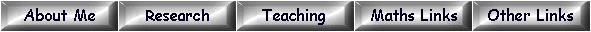Elements of order 3 in the Tate-Shafarevich group

We list elements of order 3 in the Tate-Shafarevich group for elliptic curves E over Q. To compile this data we started from Cremona's list of elliptic curves over Q with "analytic order of Sha" greater than 1. The data is available at

http://www.warwick.ac.uk/~masgaj/ftp/data/

For each elliptic curve over Q with analytic order of Sha divisible by 3 we expect to find a subgroup of Sha isomorphic to Z/3Z x Z/3Z. We have found such a subgroup in all cases up to conductor 300000 (the current limit of Cremona's tables).

The first line of each entry specifies an elliptic curve E over Q. This data is taken directly from Cremona's tables. The quantities listed are the conductor, the isogeny class, the number within the isogeny class, the coefficients [a1,a2,a3,a4,a6] of a minimal Weierstrass equation, the Mordell-Weil rank, the order of the torsion subgroup, and finally the analytic order of Sha. We then list (3k-1)/2 ternary cubics representing a subgroup of the 3-Selmer group of E isomorphic to (Z/3Z)k where k = 2 or 4 (the latter in only 14 cases so far, listed here). Each ternary cubic represents a non-zero element of the subgroup and its inverse. The coefficients are listed in the order

x3    y3    z3    x2y     x2z     y2x     y2z     z2x     z2y     xyz   .

We have checked that each subgroup of the Selmer group listed has trivial intersection with the image of the Mordell-Weil group. (In all examples so far the Mordell-Weil rank is 0 or 1.) Therefore each cubic listed is a counterexample to the Hasse Principle.

The ternary cubics listed are minimised and reduced as explained here. We have also arranged that the reduction mod p at each prime p is geometrically (i.e. over the algebraic closure of Fp) either an irreducible cubic, or the union of a non-singular conic and a line. This guarantees local solubility.

These tables were constructed using programs written in Magma by Michael Stoll, Steve Donnelly and myself, based on joint work by John Cremona, Cathy O'Neil, Denis Simon, Michael Stoll and myself.

A similar study of elements of Sha of order 5 is here.

Last updated 14th June 2013